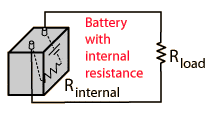# Battery with internal resistor

[SOLVED] Battery with internal resistor

## Homework Statement

What is the current in a 8.25 ohm resistor connected to a battery that has a .21 ohm internal resistance when the potential drop across the terminals of the battery is 6V?

## Homework Equations

$$R_{s}=R_{1}+R_{2}...$$

$$V=IR$$

## The Attempt at a Solution

So I believe that the internal resistor can just be considered being in series to the other resistor, allowing me to calculate the equivalent resistor. Then with that, using V=IR, I thought I was able to get the current flowing through the circuit. I got .70921 A
Can someone correct my train of thought?

Hootenanny
Staff Emeritus
Gold Member

## Homework Statement

What is the current in a 8.25 ohm resistor connected to a battery that has a .21 ohm internal resistance when the potential drop across the terminals of the battery is 6V?

## Homework Equations

$$R_{s}=R_{1}+R_{2}...$$

$$V=IR$$

## The Attempt at a Solution

So I believe that the internal resistor can just be considered being in series to the other resistor, allowing me to calculate the equivalent resistor. Then with that, using V=IR, I thought I was able to get the current flowing through the circuit. I got .70921 A
Can someone correct my train of thought?
Note that the potential drop across the terminals includes the internal resistance of the battery. The internal resistance of a battery may be depicted thus,So the actual emf of the battery is the 6V plus the voltage drop across the internal resistance. Do you follow?

Image courtesy of Hyperphysics

Last edited:

Hootenanny
Staff Emeritus
Hyperphysics is an excellent general physics reference, I'm glad that you chose to look there rather than Wikipedia